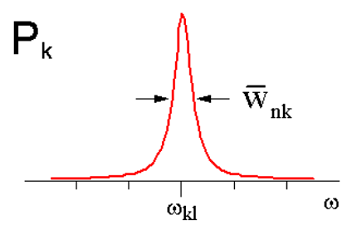$$\newcommand{\vecs}{\overset { \rightharpoonup} {\mathbf{#1}} }$$ $$\newcommand{\vecd}{\overset{-\!-\!\rightharpoonup}{\vphantom{a}\smash {#1}}}$$$$\newcommand{\id}{\mathrm{id}}$$ $$\newcommand{\Span}{\mathrm{span}}$$ $$\newcommand{\kernel}{\mathrm{null}\,}$$ $$\newcommand{\range}{\mathrm{range}\,}$$ $$\newcommand{\RealPart}{\mathrm{Re}}$$ $$\newcommand{\ImaginaryPart}{\mathrm{Im}}$$ $$\newcommand{\Argument}{\mathrm{Arg}}$$ $$\newcommand{\norm}{\| #1 \|}$$ $$\newcommand{\inner}{\langle #1, #2 \rangle}$$ $$\newcommand{\Span}{\mathrm{span}}$$ $$\newcommand{\id}{\mathrm{id}}$$ $$\newcommand{\Span}{\mathrm{span}}$$ $$\newcommand{\kernel}{\mathrm{null}\,}$$ $$\newcommand{\range}{\mathrm{range}\,}$$ $$\newcommand{\RealPart}{\mathrm{Re}}$$ $$\newcommand{\ImaginaryPart}{\mathrm{Im}}$$ $$\newcommand{\Argument}{\mathrm{Arg}}$$ $$\newcommand{\norm}{\| #1 \|}$$ $$\newcommand{\inner}{\langle #1, #2 \rangle}$$ $$\newcommand{\Span}{\mathrm{span}}$$$$\newcommand{\AA}{\unicode[.8,0]{x212B}}$$

Let’s describe absorption to a state that is coupled to a continuum. What happens to the probability of absorption if the excited state decays exponentially?$\frac {\partial} {\partial t} b _ {k} = - \frac {i} {\hbar} e^{i \omega _ {k l} t} V _ {k \ell} (t) \label{6.56}$

where we make the approximation $$b _ {\ell} (t) \approx 1$$. We can add irreversible relaxation to the description of $$b_k$$ using our earlier expression for the relaxation of

$b _ {k} (t) = \exp \left[ - \overline {w} _ {n k} t / 2 - i \Delta E _ {k} t / \hbar \right].$

In this case, we will neglect the correction to the energy $$\Delta E _ {k} = 0$$, so

$\frac {\partial} {\partial t} b _ {k} = - \frac {i} {\hbar} e^{i \omega _ {\mu l} t} V _ {k \ell} (t) - \frac {\overline {w} _ {n k}} {2} b _ {k} \label{6.57}$

Or using $$V (t) = - i E _ {0} \overline {\mu} _ {k l} \sin \omega t$$

\begin{align} \frac {\partial} {\partial t} b _ {k} & = \frac {- i} {\hbar} e^{i \omega _ {k t} t} \sin \omega t V _ {k \ell} - \frac {\overline {w} _ {n k}} {2} b _ {k} (t) \\[4pt] & = \frac {E _ {0} \omega _ {k \ell}} {2 i \hbar \omega} \left[ e^{i \left( \omega _ {k \ell} + \omega \right)} - e^{i \left( \omega _ {k \ell} - \omega \right) t} \right] \overline {\mu} _ {k \ell} - \frac {\overline {w} _ {n k}} {2} b _ {k} (t) \label{6.58} \end{align}

The solution to the differential equation

$\dot {y} + a y = b e^{i \alpha t} \label{6.59}$

is

$y (t) = A e^{- a t} + \frac {b e^{i \alpha t}} {a + i \alpha} \label{6.60}$

with

$b _ {k} (t) = A e^{- \overline {w} _ {n k} t / 2} + \frac {E _ {0} \overline {\mu} _ {k \ell}} {2 i \hbar} \left[ \frac {e^{i \left( \omega _ {k t} + \omega \right) t}} {\overline {w} _ {n k} / 2 + i \left( \omega _ {k \ell} + \omega \right)} - \frac {e^{i \left( \omega _ {k l} - \omega \right) t}} {\overline {w} _ {n k} / 2 + i \left( \omega _ {k \ell} - \omega \right)} \right] \label{6.61}$

Let’s look at absorption only, in the long time limit:

$b _ {k} (t) = \frac {E _ {0} \overline {\mu} _ {k \ell}} {2 \hbar} \left[ \frac {e^{i \left( \omega _ {k l} - \omega \right) t}} {\omega _ {k \ell} - \omega - i \overline {w} _ {n k} / 2} \right] \label{6.62}$

For which the probability of transition to $$k$$ is

$P _ {k} = \left| b _ {k} \right|^{2} = \frac {E _ {0}^{2} \left| \mu _ {k \ell} \right|^{2}} {4 \hbar^{2}} \frac {1} {\left( \omega _ {k \ell} - \omega \right)^{2} + \overline {w} _ {n k}^{2} / 4} \label{6.63}$

The frequency dependence of the transition probability has a Lorentzian form:The FWHM line width gives the relaxation rate from $$k$$ into the continuum $$n$$. Also the line width is related to the system rather than the manner in which we introduced the perturbation. The line width or line shape is an additional feature that we interpret in our spectra, and commonly originates from irreversible relaxation or other processes that destroy the coherence first set up by the light field.

This page titled 7.4: Relaxation and Line-Broadening is shared under a CC BY-NC-SA 4.0 license and was authored, remixed, and/or curated by Andrei Tokmakoff via source content that was edited to the style and standards of the LibreTexts platform; a detailed edit history is available upon request.